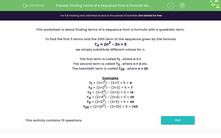# Find the Terms of a Sequence from a Formula with a Quadratic Term

In this worksheet, students will find the terms of a sequence from a T-formula that includes a quadratic term.Key stage:  KS 3

Curriculum topic:   Algebra

Curriculum subtopic:   Substitute Numerical Values for Formulae/Expressions

Difficulty level:#### Worksheet Overview

This activity is about finding terms of a sequence from a formula with a quadratic term.

To find the first 5 terms and the 20th term of the sequence given by the formula

T= 2n2 − 3n + 5

we simply substitute different values for n.

The first term is called T1 , where n = 1.

The second term is called T2 , where n = 2 etc.

The twentieth term is called T20 , where n = 20.

Examples

T1 = (2 × 12) − (3 × 1) + 5 = 4

T2 = (2 × 22) − (3 × 2) + 5 = 7

T3 = (2 × 32) − (3 × 3) + 5 = 14

T4 = (2 × 42) − (3 × 4) + 5 = 25

T5 = (2 × 52) − (3 × 5) + 5 = 40

T20 = (2 × 202) − (3 × 20) + 5 = 745

It might look a bit daunting, but just take it slowly, one step at a time and you'll soon be flying through them!

Let's get started.

### What is EdPlace?

We're your National Curriculum aligned online education content provider helping each child succeed in English, maths and science from year 1 to GCSE. With an EdPlace account you’ll be able to track and measure progress, helping each child achieve their best. We build confidence and attainment by personalising each child’s learning at a level that suits them.

Get started